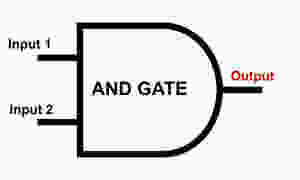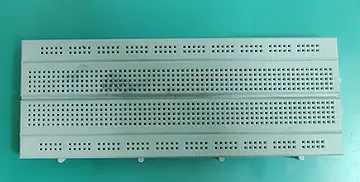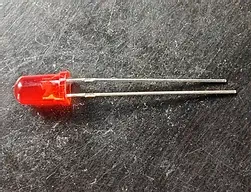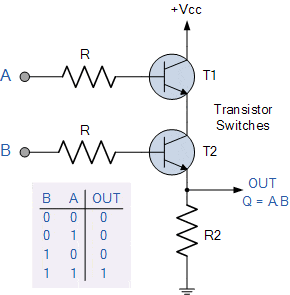top of page

# AND Gate using transistor

Updated: Apr 22, 2021

What is AND Gate?

The #logicgate that implements logical conjunction is AND Gate. The symbolic representation of AND Gate resembles D-shape. A two-input and a single output AND Gate is shown in the figure AND Gate symbolic representation. The inputs and output are connected through a Logic.AND Gate symbolic representation

The output of AND Gate is HIGH only when both the inputs are HIGH, and for the rest of all the cases, the output is LOW. This can be understood clearly from the truth table below:

A : (Input 1) B : (Input 2)O : (Output)
```   Input A        Input B        Output
0 	     0		   0
0              1		   0
1		     0             0
1	             1		   1```

The Boolean expression of an AND Gate is, O = A * B.

### How to build AND Gate using transistors?

If the output of the circuit we build has output similar to the output in the truth table above then we can conclude that AND Gate was built successfully using transistors. Now, let’s look at the list of components required for making an AND Gate using a transistor.

## Components Required for AND GATE

The list of components required to build the circuit are mentioned below:

1. Two transistors (BC 547 NPN transistors).BC 547 Transistor for AND GATE

BC 547 NPN is a low-power amplifying transistor which is a common #NPN Bipolar Junction transistor (#BJT). In AND GATE the transistor is usually used for Switching purposes as it is used in our circuit.

2. One power supply(5V preferable).Power Supply Module

In making AND GATE using transistor, the voltage supply to circuits designed with components on a small scale is provided by the Power Supply module . The module has a Barrel jack that can be connected to the DC supply directly. The Voltage is regulated to supply 6V to12 V DC. The regulated voltage is then supplied to the circuit.

The power channel can be configured to supply voltages of 0V, 3.3V, or 5V. A control button that can operate the circuit as per our requirement, i.e., to start and stop the power supply is present. The power is supplied through the module is indicated by an onboard LED. The power supply for this circuit can be 5V or 6V.

3. 10K Ohm resistors (2) and 5K Ohm resistor (1).10k Ohm Resistor5k Ohm Resistor.

Resistors are devices that oppose the flow of electrons. The resistance value of resistors used in this circuit are 10k Ohm and 5k Ohm. 10k Ohm Resistor is connected in the circuit before the transistor, i.e. the input power passes through the resistors and then to the transistors. The 5k Ohm resistor is connected before the LED so that the voltage diverted to the LED will be less.The breadboard is the basic component used in the circuit-building process. All components in the circuit are connected using wired connections through a breadboard. The holes in the breadboard are connected in series. They are available in different sizes like full-sized, half-sized, and mini breadboards.

5. Connecting Wires.Connecting Wires

The Connecting Wires are thin components used to establish the connections between different devices of the circuit.

6. One LED(To see the output)LED

Light Emitting Diode (LED) is a commonly used light source. LED is a semiconductor that emits light when the current passes through it.

7. Two Push ButtonsPush Button

PUSH Buttons are devices used for switching control. It is easy to use and push-button is used to connect or disconnect the component(s) in the circuit (Wires can be used as an alternative).

## Circuit Diagram of AND GATE

Using all the above-mentioned components, a hardware circuit is built that is shown in the below hardware schematic. Now, let's understand the design and working of AND Gate using a transistor.AND Gate Schematic Diagram

The hardware schematic shown above resembles the circuit diagram. In this circuit, 5V (VCC) power supply is connected to the collector terminal of the first transistor (T1) directly. Both the transistors, T1 and T2 are connected in serial with each other same as the NAND Gate circuit and also the circuit somewhat resembles the NAND gate circuit built using transistors.

The base terminal of both the transistors T1 and T2 are connected to inputs A and B, respectively through a 10K Ohm resistor for each resistor viz. R & R, and finally, the emitter terminal of the transistor T2 is connected directly to the ground using a resistor of resistance 5K Ohm (R2). To check the output of the circuit, the emitter terminal of the transistor, T2 is connected with the output LED which in turn is connected to the ground to complete the circuit.AND Gate Circuit Diagram

## Working of AND GATE using transistor

The working of the AND gate circuit can be well understood by considering the 4 cases from the truth table. So, if the output from the hardware and the output of the truth table matches for each case of the inputs then we can say that we have successfully built an AND Gate using transistors. Now, let’s see all the 4 cases in AND GATE one by one:

Case 1 : Input A = 0 and Input B = 0.

When inputs A and B are 0, then output (O) should also be 0, according to the AND Gate Boolean expression i.e. output, O = A * B. This can be proved when we see the result of the hardware circuit.AND GATE using transistor

In the circuit, the 5V power supply passes to the #collector terminal but since the input at the base of transistor T1 is 0, there is no connection between the collector and emitter of the transistor T1.

So, the current does not pass from the collector terminal of transistor T1. Now the output is connected to the emitter terminal of the second transistor and the output is zero. As a result, the LED connected to the #emitter terminal of transistor will be in OFF state.

Case 2 : Input A = 0 and Input B = 1.

For inputs A = 0 and B = 1, the output according to the Boolean expression of AND Gate is 0. As a result, the LED will be in OFF condition.CASE 2: Input A=0 and B=1

In the circuit, when a supply of 5V is connected to the collector terminal of transistor T1 and if input A connected to the base of the same transistor is 0 then, the electrical signal will not pass from the collector terminal of the transistor to the emitter terminal of transistor T1. So, the collector of the transistor has LOW input and the same would pass to the emitter terminal since the #base of transistor T2 connected with B has HIGH input. As a result, the emitter would pass the LOW value to the LED and the LED will be in OFF state.

Case 3 : Input A = 1 and Input B = 0.

Here, the working is a bit opposite to the previous case i.e. the current would pass through the transistor T1 but not through the #transistor T2, being the input A = 1 and B = 0. So, the current would pass only till the collector terminal of transistor T2 but not to the emitter of transistor T2. The emitter of T2 has a LOW value and the LED will have a LOW input. Therefore, the LED will be in an OFF state.CASE 3 Input A=1 and B=0

According to the Boolean expression of AND Gate i.e. output, O = A * B, and when input A = 1 and B = 0, the output will be 0.

Case 4 : Input A = 1 and Input B = 1.

As mentioned before, for an AND Gate the output is HIGH only when both the inputs are HIGH and so explains the #boolean expression. If both the inputs A and B are 1 then the output (O = A * B) will be 1.CASE 4 Input A=1 and B=1

In the hardware circuit (AND GATE using transistor) when both the inputs A and B are HIGH, the base terminal for both the transistors allows a connection between the collector and emitter of both transistors. Thus, a path is created from the 5V supply to the emitter terminal of transistor T2 via transistor T1 and hence, the 5V supply reaches the output terminal making the LED turn ON.

You can try practically by watching the video and understand the working of AND Gate using transistor.

Author -

Written By: LearnElectronics India

Modified By: Nagapuri Swathi